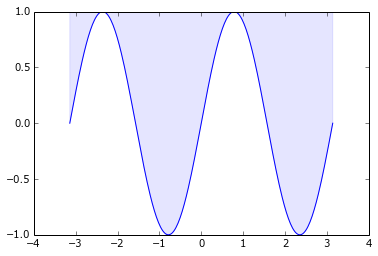## Matplotlib Tutorial

### Introduction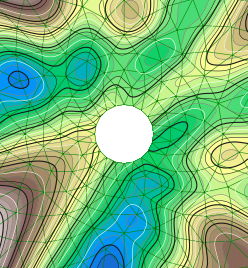Matplotlib is a plotting library like GNUplot. The main advantage towards GNUplot is the fact that Matplotlib is a Python module. Due to the growing interest in python the popularity of matplotlib is continually rising as well.

Another reason for the attractiveness of Matplotlib lies in the fact that it is widely considered to be a perfect alternative to MATLAB, if it is used in combination with Numpy and Scipy. Whereas MATLAB is expensive and closed source, Matplotlib is free and open source code. It is also object-oriented and can be used in an object oriented way. Furthermore it can be used with general-purpose GUI toolkits like wxPython, Qt, and GTK+. There is also a procedural "pylab", which designed to closely resemble that of MATLAB. This can make it extremely easy for MATLAB users to migrate to matplotlib.

Matplotlib can be used to create publication quality figures in a variety of hardcopy formats and interactive environments across platforms.

Another characteristic of matplotlib is its steep learning curve, which means that users usually make rapid progress after having started. The officicial website has to say the following about this: "matplotlib tries to make easy things easy and hard things possible. You can generate plots, histograms, power spectra, bar charts, errorcharts, scatterplots, etc, with just a few lines of code."

### A First Example

We will start with a simple graph , which is as simple as simple can be. A graph in matplotlib is a two- or three-dimensional drawing showing a relationship by means of points, a curve, or amongst others a series of bars. We have two axis: The horizontal X-axis is representing the independent values and the vertical Y-axis corresponds to the depended values.

We will use the pyplot submodule of matplotlib. pyplot provides a procedural interface to the object-oriented plotting library of matplotlib.
Its plotting commands are chosen in a way that they are similar to Matlab both in naming and with the arguments.

Is is common practice to rename matplotlib.pyplot to plt. We will use the plot function of pyplot in our first example. We will pass a list of values to the plot function. Plot takes these as Y values. The indices of the list are automatically taken as the X values. The command %matplotlib inline makes only sense, if you work with Ipython Notebook. It makes sure, that the graphs will be depicted inside of the document and not as independent windows:

%matplotlib inline

import matplotlib.pyplot as plt
plt.plot([-1, -4.5, 16, 23])
plt.show()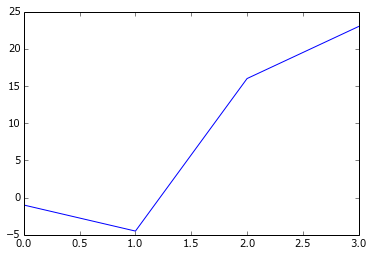What we see is a continuous graph, even though we provided discrete data for the Y values. By adding a format string to the function call of plot, we can create a graph with discrete values, in our case blue circle markers. The format string defines the way how the discrete points have to be rendered.

import matplotlib.pyplot as plt
plt.plot([-1, -4.5, 16, 23], "ob")
plt.show()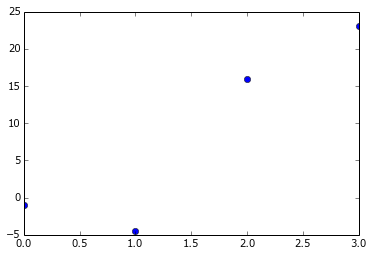### The format parameter of pyplot.plot

We have used "ob" in our previous example as the format parameter. It consists of two characters. The first one defines the line style or the dicrete value style, i.e. the markers, while the second one chooses a colour for the graph. The order of the two characters could have been reversed, i.e. we could have written it as "bo" as well. If the format parameter is not giving, as it has been the case in our first example, "b-" is used as the default value, i.e. a solid blue line.

The following format string characters are accepted to control the line style or marker:

=============================================
character       description
=============================================
'-'             solid line style
'--'            dashed line style
'-.'            dash-dot line style
':'             dotted line style
'.'             point marker
','             pixel marker
'o'             circle marker
'v'             triangle_down marker
'^'             triangle_up marker
'<'             triangle_left marker
'>'             triangle_right marker
'1'             tri_down marker
'2'             tri_up marker
'3'             tri_left marker
'4'             tri_right marker
's'             square marker
'p'             pentagon marker
'*'             star marker
'h'             hexagon1 marker
'H'             hexagon2 marker
'+'             plus marker
'x'             x marker
'D'             diamond marker
'd'             thin_diamond marker
'|'             vline marker
'_'             hline marker
===============================================


The following color abbreviations are supported:

==================
character   color
==================
'b'         blue
'g'         green
'r'         red
'c'         cyan
'm'         magenta
'y'         yellow
'k'         black
'w'         white
==================

As you may have guessed already, you can X values to the plot function. We will pass the multiples of 3 up 21 including the 0 to plot in the following example:

import matplotlib.pyplot as plt
# our X values:
days = list(range(0, 22, 3))
print(days)
# our Y values:
celsius_values = [25.6, 24.1, 26.7, 28.3, 27.5, 30.5, 32.8, 33.1]
plt.plot(days, celsius_values)
plt.show()

[0, 3, 6, 9, 12, 15, 18, 21]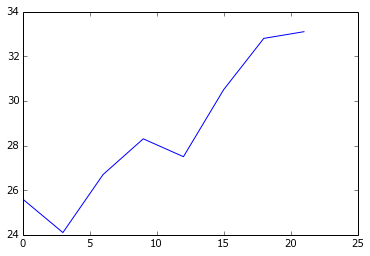... and once more with discrete values:

plt.plot(days, celsius_values, 'bo')
plt.show()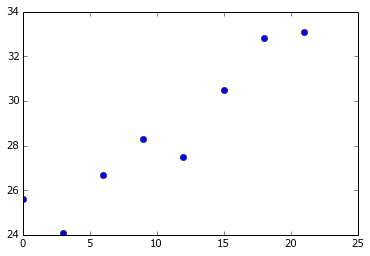### Labels on Axes

We can improve the appearance of our graph by adding labels to the axes. This can be done with the ylabel and xlabel function of pyplot.

import matplotlib.pyplot as plt
days = list(range(1,9))
celsius_values = [25.6, 24.1, 26.7, 28.3, 27.5, 30.5, 32.8, 33.1]
plt.plot(days, celsius_values)
plt.xlabel('Day')
plt.ylabel('Degrees Celsius')
plt.show()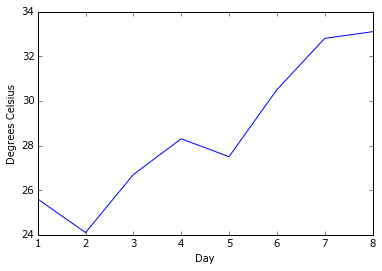We can specify an arbitrary number of x, y, fmt groups in a plot function. In the following example, we use two different lists of y values:

import matplotlib.pyplot as plt
days = list(range(1,9))
celsius_min = [19.6, 24.1, 26.7, 28.3, 27.5, 30.5, 32.8, 33.1]
celsius_max = [24.8, 28.9, 31.3, 33.0, 34.9, 35.6, 38.4, 39.2]
plt.xlabel('Day')
plt.ylabel('Degrees Celsius')
plt.plot(days, celsius_min,
days, celsius_min, "oy",
days, celsius_max,
days, celsius_max, "or")
plt.show()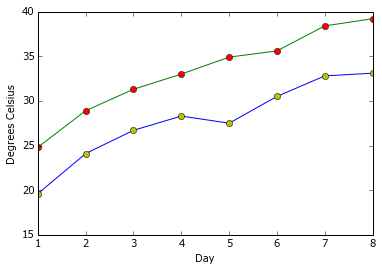### Checking and Defining the Range of Axes

We can also check and define the range of the axes with the function axis. If you call it without arguments it returns the current axis limits:

days = list(range(1,9))
celsius_min = [19.6, 24.1, 26.7, 28.3, 27.5, 30.5, 32.8, 33.1]
celsius_max = [24.8, 28.9, 31.3, 33.0, 34.9, 35.6, 38.4, 39.2]
plt.xlabel('Day')
plt.ylabel('Degrees Celsius')
plt.plot(days, celsius_min,
days, celsius_min, "oy",
days, celsius_max,
days, celsius_max, "or")
print("The current limits for the axes are:")
print(plt.axis())
print("We set the axes to the following values:")
xmin, xmax, ymin, ymax = 0, 10, 14, 45
print(xmin, xmax, ymin, ymax)
plt.axis([xmin, xmax, ymin, ymax])
plt.show()

The current limits for the axes are:
(1.0, 8.0, 15.0, 40.0)
We set the axes to the following values:
(0, 10, 14, 45)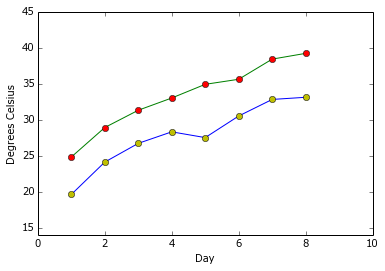import matplotlib.pyplot as plt
days = list(range(1,9))
celsius_min = [19.6, 24.1, 26.7, 28.3, 27.5, 30.5, 32.8, 33.1]
celsius_max = [24.8, 28.9, 31.3, 33.0, 34.9, 35.6, 38.4, 39.2]
plt.xlabel('Day')
plt.ylabel('Degrees Celsius')
plt.plot(days, celsius_min,
days, celsius_min, "oy",
days, celsius_max,
days, celsius_max, "or")
plt.axis([0, 10, 18, 41])
plt.show()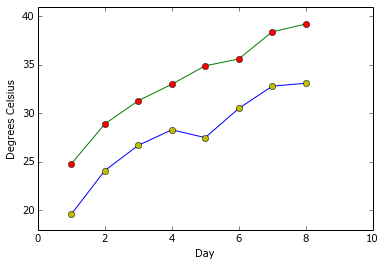### "linspace" to Define X Values

We will use the Numpy function linspace in the following example. linspace can be used to create evenly spaced numbers over a specified interval.

import numpy as np
import matplotlib.pyplot as plt
X = np.linspace(0, 2 * np.pi, 50, endpoint=True)
F = np.sin(X)
plt.plot(X,F)
startx, endx = -0.1, 2*np.pi + 0.1
starty, endy = -1.1, 1.1
plt.axis([startx, endx, starty, endy])
plt.show()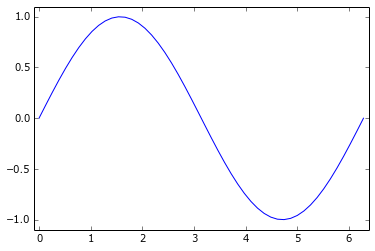import matplotlib.pyplot as plt
X = np.linspace(-2 * np.pi, 2 * np.pi, 50, endpoint=True)
F1 = 3 * np.sin(X)
F2 = np.sin(2*X)
F3 = 0.3 * np.sin(X)
startx, endx = -2 * np.pi - 0.1, 2*np.pi + 0.1
starty, endy = -3.1, 3.1
plt.axis([startx, endx, starty, endy])
plt.plot(X,F1)
plt.plot(X,F2)
plt.plot(X,F3)
plt.show()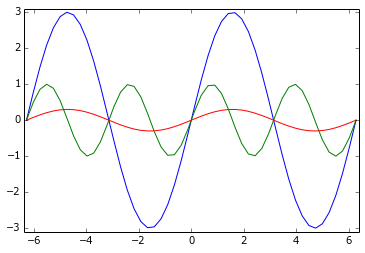The next example isn't anything new. We will just add two more plots with discrete points:

import matplotlib.pyplot as plt
X = np.linspace(-2 * np.pi, 2 * np.pi, 50, endpoint=True)
F1 = 3 * np.sin(X)
F2 = np.sin(2*X)
F3 = 0.3 * np.sin(X)
startx, endx = -2 * np.pi - 0.1, 2*np.pi + 0.1
starty, endy = -3.1, 3.1
plt.axis([startx, endx, starty, endy])
plt.plot(X,F1)
plt.plot(X,F2)
plt.plot(X,F3)
plt.plot(X, F1, 'ro')
plt.plot(X, F2, 'bx')
plt.show()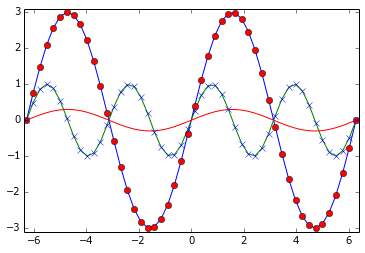### Changing the Line Style

The linestyle of a plot can be influenced with the linestyle or ls parameter of the plot function. It can be set to one of the following values:
'-', '--', '-.', ':', 'None', ' ', ''

We can use linewidth to set the width of a line as the name implies.

import matplotlib.pyplot as plt
X = np.linspace(0, 2 * np.pi, 50, endpoint=True)
F1 = 3 * np.sin(X)
F2 = np.sin(2*X)
F3 = 0.3 * np.sin(X)
F4 = np.cos(X)
plt.plot(X, F1, color="blue", linewidth=2.5, linestyle="-")
plt.plot(X, F2, color="red", linewidth=1.5, linestyle="--")
plt.plot(X, F3, color="green", linewidth=2, linestyle=":")
plt.plot(X, F4, color="grey", linewidth=2, linestyle="-.")
plt.show()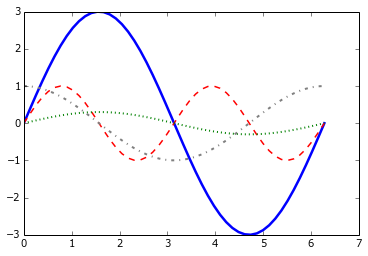It is possible to shade or colorize regions between two curves. We are filling the region between the X axis and the graph of sin(2*X) in the following example:

import numpy as np
import matplotlib.pyplot as plt
n = 256
X = np.linspace(-np.pi,np.pi,n,endpoint=True)
Y = np.sin(2*X)
plt.plot (X, Y, color='blue', alpha=1.00)
plt.fill_between(X, 0, Y, color='blue', alpha=.1)
plt.show()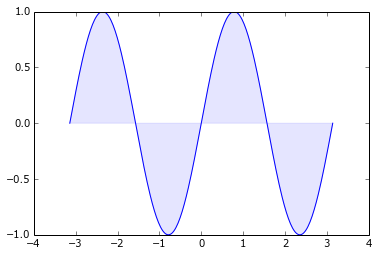The general syntax of fill_between:

fill_between(x, y1, y2=0, where=None, interpolate=False, **kwargs)

The parameters of fill_between:

Parameter Meaning
x An N-length array of the x data
y1 An N-length array (or scalar) of the y data
y2 An N-length array (or scalar) of the y data
where If None, default to fill between everywhere. If not None, it is an N-length numpy boolean array and the fill will only happen over the regions where where==True.
interpolate If True, interpolate between the two lines to find the precise point of intersection. Otherwise, the start and end points of the filled region will only occur on explicit values in the x array.
kwargs Keyword args passed on to the PolyCollection
import numpy as np
import matplotlib.pyplot as plt
n = 256
X = np.linspace(-np.pi,np.pi,n,endpoint=True)
Y = np.sin(2*X)
plt.plot (X, Y, color='blue', alpha=1.00)
plt.fill_between(X, Y, 1, color='blue', alpha=.1)
plt.show()